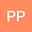Numerical solution of a class of two-dimensional non-linear variable order fractional reaction-diﬀusion equations in porous media
•• Prashant Pandey
Prashant Pandey
Indian Institute of Technology Banaras Hindu University

Corresponding Author:[email protected]

Author Profile## Abstract

In the present scientiﬁc article, an eﬃcient operational matrix based on the famous Bernstein polynomials is applied for the numerical solution of two-dimensional non-linear variable order reaction-diﬀusion equation in porous media with given initial and boundary conditions. An operational matrix is constructed for fractional variable order diﬀerentiation w.r.to space variable x,y and time t, so that our proposed model is converted into a system of non-linear algebraic equations with the help of collocation method, which can be solved employing the Newton-Iteration method. The salient features of the article are ﬁnding the stability analysis and error bounds of the proposed method and also the validation and the eﬀectiveness of the method through the RMS, L∞ and L2 errors. The physical presentation of the these errors for considered twodimensional non-linear variable order reaction-diﬀusion with their exact solutions shows the method is too good for ﬁnding the solution of these kind of problems.